# A Unified Theory of Garbage Collection

## Summary

Tracing and reference counting are normally viewed as the two main, completely different approaches to garbage collection. However, in A Unified Theory of Garbage Collection, Bacon et al. showed tracing and reference counting to be duals of one another, and that all garbage collectors are various types of hybrids of tracing and reference counting. Intuitively, tracing is tracking the live objects while reference counting is tracking dead objects.

## Background

Broadly speaking, garbage collection (GC) is a form of automatic memory management. The garbage collector attempts to free the memory blocks occupied by objects that are no longer in use by the program. It relieves programmers from the burden of explicitly freeing allocated memory. Moreover, it also serves as part of the security strategy of languages like Java: in the Java virtual machine programmers are unable to accidentally (or purposely) crash the machine by incorrectly freeing memory. The opposite is manual memory management, which is available in C/C++. This gives the maximum freedom for programmers and avoids the potential overhead that affects program performance.

The task garbage collection needs to solve is identifying the objects not accessible by the program in the reference graph. It then frees the unreachable objects and rearranges the memory sometimes to reduce heap fragmentation.

The most traditional approaches are tracing and reference counting:

• Tracing: Recursively mark reachability by starting from a set of root memory blocks that are in use (e.g., pointed to by global variables or local variables currently in stack frames).
• Reference Counting: Count the number of pointers pointing to one particular object by bookkeeping it every time a pointer is created or modified. It frees the object when the counter decreases to zero.

These two approaches have a lot differences: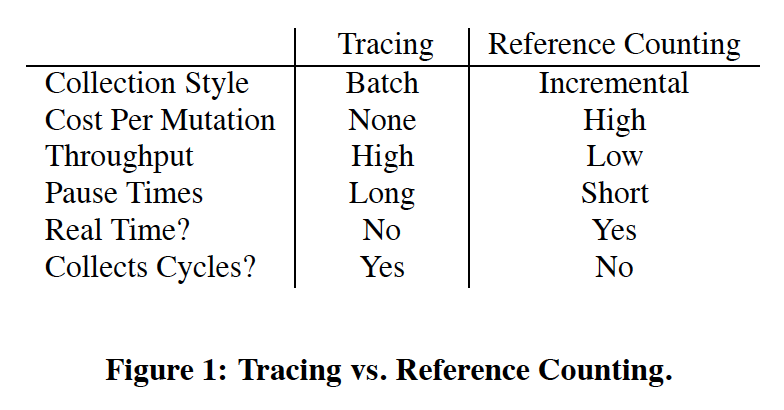Although tracing naturally solves the reachability problem accurately, it requires traversing over a static graph and therefore suspending the whole program. On the other hand, reference counting is done incrementally along with each pointer assignment. However, it brings unnecessary overhead when the pointers are changed often and it does not collect cycles of garbage. Thus people proposed more complicated algorithms based on different hypotheses, such as deferred reference counting, generational garbage collection, etc.

## Tracing & Reference Counting are Duals

On the high level, tracing is tracking "matter" -- all reachable objects, while reference counting is tracking "anti-matter" -- all unreachable objects. Their connection is further revealed when we align them by removing certain "optimizations". We can consider a version of tracing that computes the number of incoming edges from roots or live objects instead of a single bit; and a version of reference counting that postpones the decrements to be processed in batches. If the graph contains no cycles, both methods would converge to tagging the same value for each object. Tracing achieves this by setting this value to zero and increases it recursively, while reference counting starts from an upper bound and decrements it recursively.

To formalize this connection, we define the value they converge to mathematically then align their algorithmic structures.

### Mathematical Model

In order to analyze and compare different garbage collection strategies, the paper presents a mathematical model of the memory management problem that garbage collection is trying to solve. Objects in memory and the pointers that they contain are modeled as the nodes and edges of a graph, respectively. The set of objects is denoted as $V$, and the multiset of pointers between objects is $E$. An object should not be freed if it will be used in the future. A conservative approximation of this, without any program analysis, is that an object might be used in the future if there exists a path of pointers to the object which originates from the stack or from a register. We call the starting points of such paths (i.e., all objects to which there is a direct pointer on the stack or in a register) the roots of the graph, which make up the multiset $R$.

Using these definitions, we can formulate the reference counts of objects (denoted $\rho(v)$ for $v \in V$) as a fixed point of the following equation:

$$\rho(v) = \big|[v : v \in R]\big| + \big|[(w, v) : (w, v) \in E \land \rho(w) > 0]\big|$$

Here we recursively define the reference count of an object $v$ to be the number of root pointers to $v$ plus the number of pointers to $v$ from objects which themselves have non-zero reference counts. Any object whose reference count is zero according a fixed point of $\rho$ can be freed, as there is no way for the program to reference it in the future.

However, it is important to note that there could be multiple fixed points to this equation, namely in the presence of cyclic garbage. If object $a$ points to object $b$, and vice versa, but neither is a root and neither is pointed to from elsewhere, then either $\rho(a) = \rho(b) = 1$ or $\rho(a) = \rho(b) = 0$ is a valid solution to the fixed-point equation. Ideally, a garbage collection algorithm will find the least fixed point of $\rho$, meaning that it will consider all cyclic garbage as able to be freed. A tracing collector does this, whereas a reference counting collector does not detect any cyclic garbage, and thus finds the greatest fixed point.

### Alignments of Algorithmic Structures

As modeled in the paper, a tracing garbage collector fundamentally works by performing a traversal on the memory graph, starting from the root objects in $R$. Every time it reaches an object, it increments its reference count. And, when it reaches an object for the first time, it traverses the pointers from that object. In order to complete the traversal, the algorithm maintains a work list, $W$, of objects that it needs to visit. This traversal recomputes all reference counts completely each time it runs. When it terminates, every live object will have a positive reference count, and every dead object will have a reference count of zero.

This formulation of the algorithm differs in a few ways from a standard tracing collector. For example, there is no need in a real tracing collector to keep track of the full reference count of each object; it is sufficient to keep just a single mark bit which determines whether the reference count is non-zero. A tracing collector might also employ other optimizations, such as pointer reversal, to improve its run time or memory usage. However, these optimizations do not significantly change the core ideas of the algorithm.

Reference counting collection differs from tracing collection in that the reference counts persist over time and are computed iteratively. The paper models reference counting collectors to keep a work list, just as tracing collectors do. Every time a pointer is stored somewhere, its reference count is immediately incremented. Every time a pointer is overwritten or erased, it is added to the work list. Then, when the collection algorithm runs, the work list is iterated through, decrementing the reference count of each object in the list, and, if this causes the reference count to become zero, adding any objects that are pointed to by that object to the work list. When this algorithm terminates, every live object will still have a positive reference count, and the reference counts of dead objects could be zero (depending on if they are part of a garbage cycle).

Real implementations of reference counting collection will typically not have a work list. Instead, they will immediately decrement the reference count whenever a pointer is overwritten, and, if the reference count becomes zero, recursively decrement the reference count of anything that is pointed to by that object. The algorithm is presented in this work-list format in order to more clearly represent its relationship to tracing garbage collection. Formulated in this way, if we look at the code for the central work-list algorithms for each type of garbage collector, we can see striking similarities between them: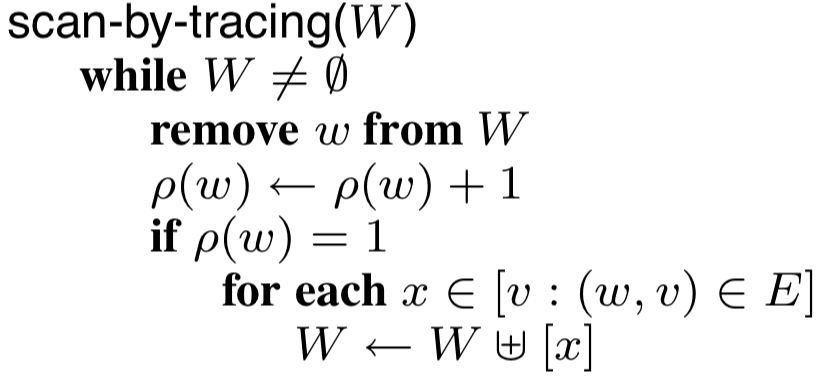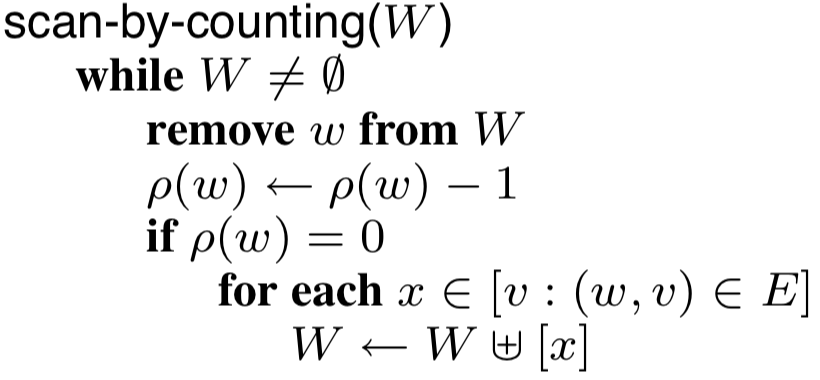Graph traversal algorithm for tracingWork-list algorithm for RC

The only differences between the two are that tracing increments the reference counts of objects on the worklist whereas RC decrements them, and that tracing checks if $\rho(w) = 1$ for when to add the objects that $w$ points to whereas RC checks if $\rho(w) = 0$. The two garbage collection strategies also differ in how $W$ is initialized. For tracing, $W$ initially contains all of the roots of the graph, $R$. For reference counting, $W$ starts with all of the objects that have had a pointer to them erased since the last time the algorithm ran. By viewing the two strategies through this formulation, we can see that they are opposites of each other in many ways: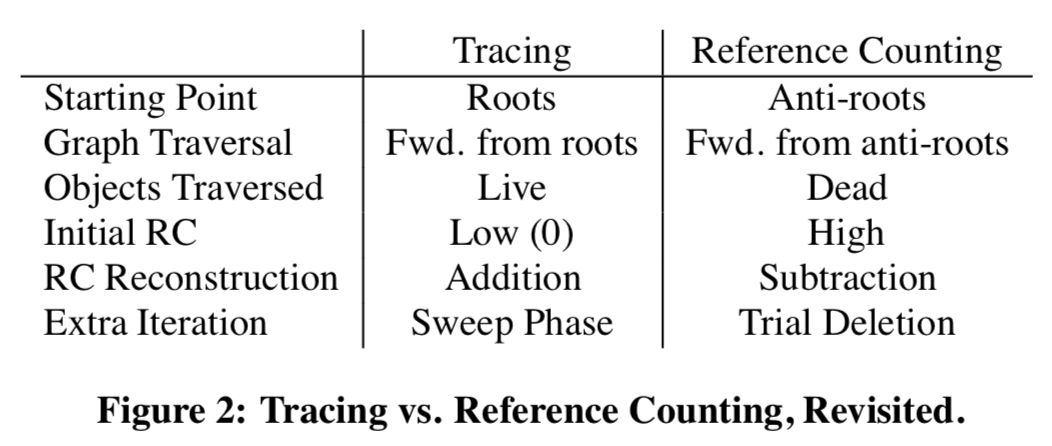As discussed in the mathematical model part, reference counting requires an extra pass to collect cycles. This is generally be done by two strategies: backup tracing, which traces (part of) the heap occasionally, and trial deletion, which attempts to decrease $\rho(w)$ by trial-and-error guided by heuristics. However, notice that there is also a counterpart for that in tracing: the sweeping phase. In addition, while tracing converges to the fixed point value starting from the lower bound of all reference counts at 0, reference counting starts from the upper bound, which contains all incoming pointers that existed at the time of the previous collection.

## Hybrids

The authors further show that all realistic garbage collectors are in fact hybrids of tracing and reference counting. In general, we can categorize collectors to unified heap collectors, split heap collectors, and multi-heap collectors. Then different garbage collectors can be seen as performing tracing or reference counting when tracking references within each region and across regions.

### Unified Heap collectors: Deferred Reference Counting & Partial Tracing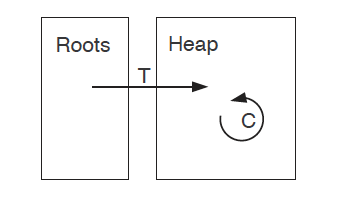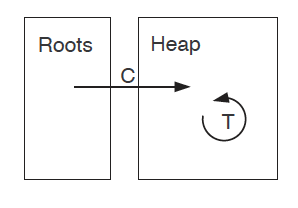Deferred Reference CountingPartial Tracing

Rather than doing reference counting completely, Deferred Reference Counting defers updating the reference counts of objects pointed to directly by roots until batch processing. This is based on the observation that pointers from roots are likely to change very often as they are directly used in the program. Notice that we can view this as tracing from roots to their targets and reference counting for the intra-heap pointers: All the assignments that lead to intra-heap pointer changes would be tracked by reference counting as normal. When we suspend the program, we trace the roots for one level, which compensates for the delay.

Reversely, we could design Partial Tracing, which uses reference counting for edges from roots to heaps while tracing the intra-heap pointers. However, this combines the worst properties of both tracing and reference counting: it suffers from the high mutation cost from the fast-changing of root pointers while still need to spend a long time to trace the heap. This design failure demonstrates that although tracing and reference counting are duals, they are not equally easy to solve under different cases.

### Split-Heap and Multi-Heap Collectors

The most common split-heap collector architecture is generational collectors. Generational collectors are based on the empirical observation that most objects are short lived, as shown in the left figure below. Here the Y axis shows the number of bytes allocated and the X axis shows the number of bytes allocated over time.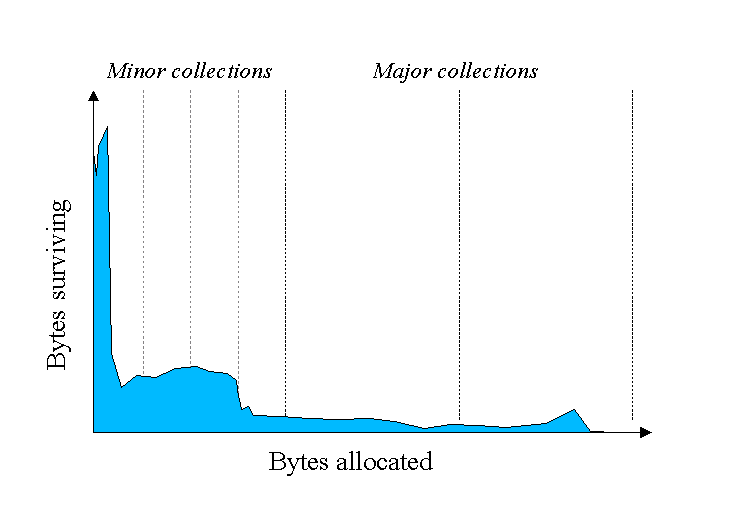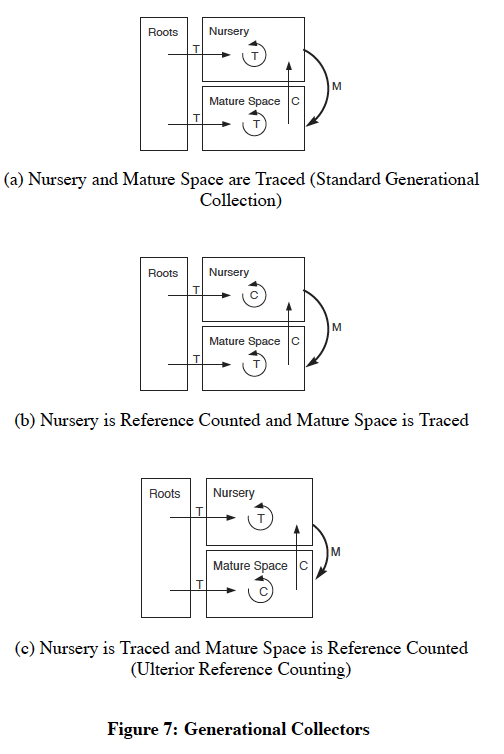Most objects are short livedGenerational collectors

So a generational collector isolates out a nursery space from the remaining mature space. Most of the time it only collects garbage from this nursery space and moves the remaining alive objects to mature space (minor collections). Once in a while it performs a garbage collection across the whole heap to clean the mature space (major collections). An example of generational collectors is available in this blog.

This process can also be seen as a combination of tracing and reference counting as shown by (a) in the right figure: Reference counting is performed to track edges from mature space to nursery space. Tracing is performed within nursery space during minor collections. And finally a full tracing is performed during major collections.

We can then explore different combinations of tracing and reference counting within each space. However, notice that reference counting is always used for the edges from mature to nursery space in order to avoid visiting all objects in the mature space. In fact, the authors claim that any algorithm that collects some subset of objects independently is fundamentally making use of reference counting.

This conclusion is further used for multi-heap collectors, which split heaps into multiple regions and collect each region individually. They can be treated asymmetrically (as in generational collectors) or symmetrically. One of the basic extensions from generational collectors is the Train algorithm. The Train algorithm extended the mature space to multiple regions of different "generations", where only one region is collected at a time. This is mainly for reducing the pause time for collecting the mature space. Specifically, it divides the Mature Object Space (MOS) to cars of fixed size, which are chained to form trains. Each time, the current youngest car will be collected, until the whole first train is collected. We assume that then this process moves on to the next train, but then it is unclear to us why we need several trains instead of one train with all cars?

## Cost Analysis

The paper also presents an abstract mathematical representation for analyzing and comparing the space and time costs of different garbage collectors. In order to represent the memory that a garbage collector requires, they define $\sigma(X)$, for any garbage collector $X$, to be the space overhead of $X$ in units of the size of a single object. For several of the garbage collectors discussed in the paper, they give equations or approximations of $\sigma$. As an example, for a tracing collector, $\sigma(T) \simeq \frac{\mathcal{M}}{\omega} + \mathcal{D}$, where $\mathcal{M}$ is the capacity of memory in units of the object size, $\omega$ is the size of an object in bits, and $\mathcal{D}$ is the space cost of the traversal stack, proportional to the traversal depth. This model represents a version of tracing collection without pointer reversal, and hence includes the traversal stack. If pointer reversal were considered, $\mathcal{D}$ could be removed from the space equation, but this would also increase the time cost of the collector due to the additional graph traversal required.

They define $\tau(X)$ to be the time overhead of a garbage collector. For each collector, they define $\tau$ in terms of a linear function of various properties of the program, omitting the constant factors that could vary between implementations. In general, they define the overhead as $\tau(X) = \phi(X)\kappa(X) + \mu(X)$, where $\phi$ is the frequency at which collection occurs, $\kappa$ is the run time of a single garbage collection execution, and $\mu$ is the overhead for mutation in the program (e.g., incrementing and decrementing reference counts). Each of these terms depends on the type of garbage collection, as well as the program that the collection is running on, and is defined more specifically in the paper for several collection strategies.

These equations for the time and space costs of various collectors in terms of parameters of the workload enable the comparison of different collectors to determine how well-suited they are for certain mutator programs and constraints. While not giving the exact formulations for these properties, which would be difficult to do in general, the abstracted equations give an idea of how the space and time of a collector vary with their parameters, and thus what the important constraints of each are.

## Conclusion

By better understanding the shared structure and duality of tracing and reference counting collectors, we can consider the design of a high-performance garbage collector as a balance between the two base strategies. And, by modeling the time and space costs of different collectors, we can formally analyze the trade-offs between different collection strategies for a specific workload or in general. The paper suggests that the design of a garbage collector involves three major decisions: how to partition memory, how to traverse memory, and what space-time trade-offs should be used. The heap can be divided into multiple segments, as with generational and multi-heap collectors, or can be collected uniformly. Each component of the heap, as well as the roots of the graph, can then be traversed and collected through either tracing or reference counting. And within the implementation of the two strategies there are other space-time trade-offs to consider as well, such as whether to employ pointer reversal.

In general, this paper provides a beautiful perspective that unifies garbage collectors to a spectrum between tracing and reference counting. It affects how garbage collectors are viewed afterward and the development of other unified theories in systems.

However, there are several aspects ignored in this paper too. Note that in this paper, the authors are only concerned with identifying unreachable objects correctly with high performance in terms of speed and space usage. This assumes that malloc can always do a perfect job to avoid memory fragmentation. But some garbage collectors are also designed to compact objects (i.e., copying garbage collectors).

In addition, this formulation also ignores reachable memory leaks fundamentally. Although the memory manager can recover unreachable memory, it cannot free memory that is still reachable and therefore potentially still useful. Modern memory managers therefore provide techniques for programmers to semantically mark memory with varying levels of usefulness, which correspond to varying levels of reachability.

Finally, there are also other methods that do automatic memory management partially in/before compile time: abstract garbage collection, which soundly over-approximates the behaviour of a concrete interpreter and self-collecting mutators, which imposes extra invariants to help garbage collection.